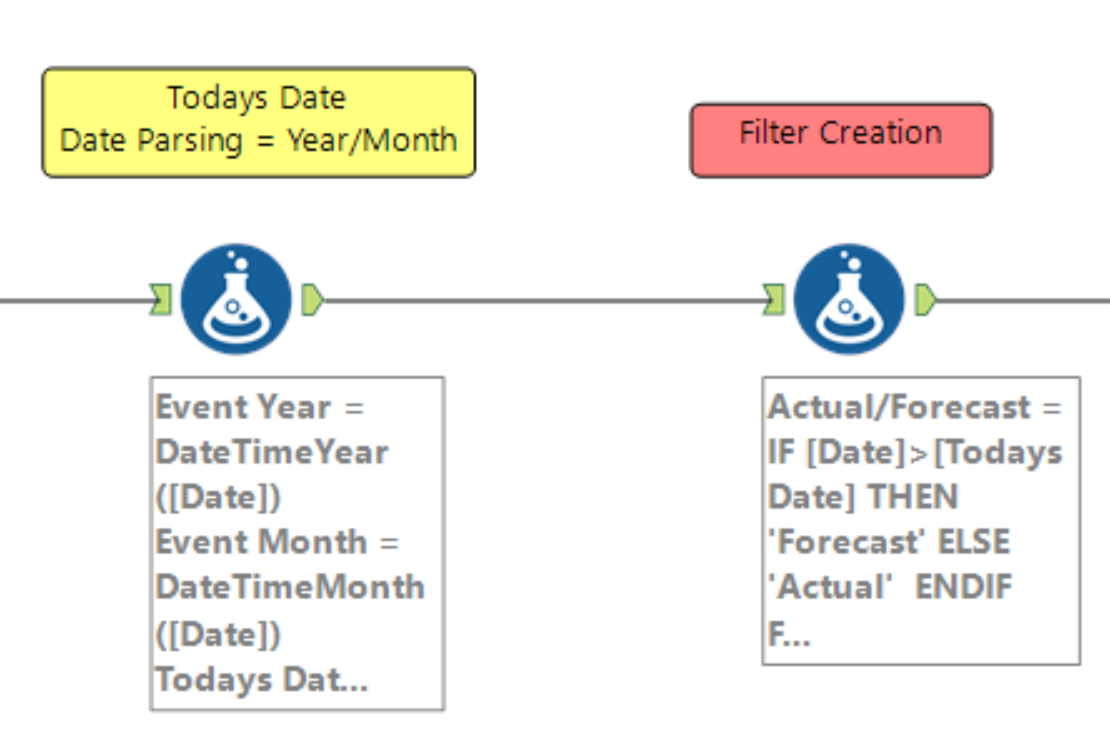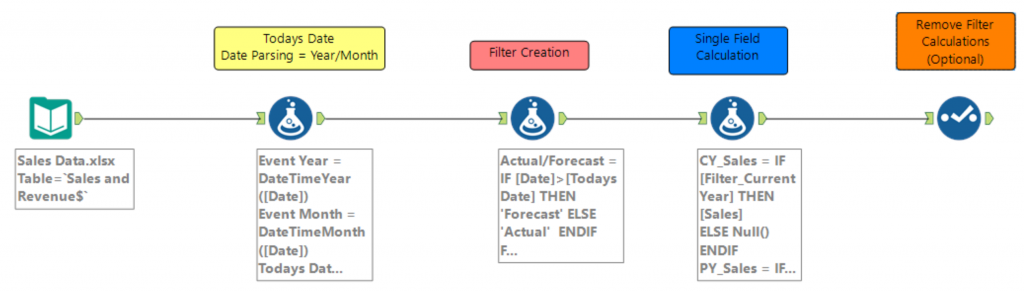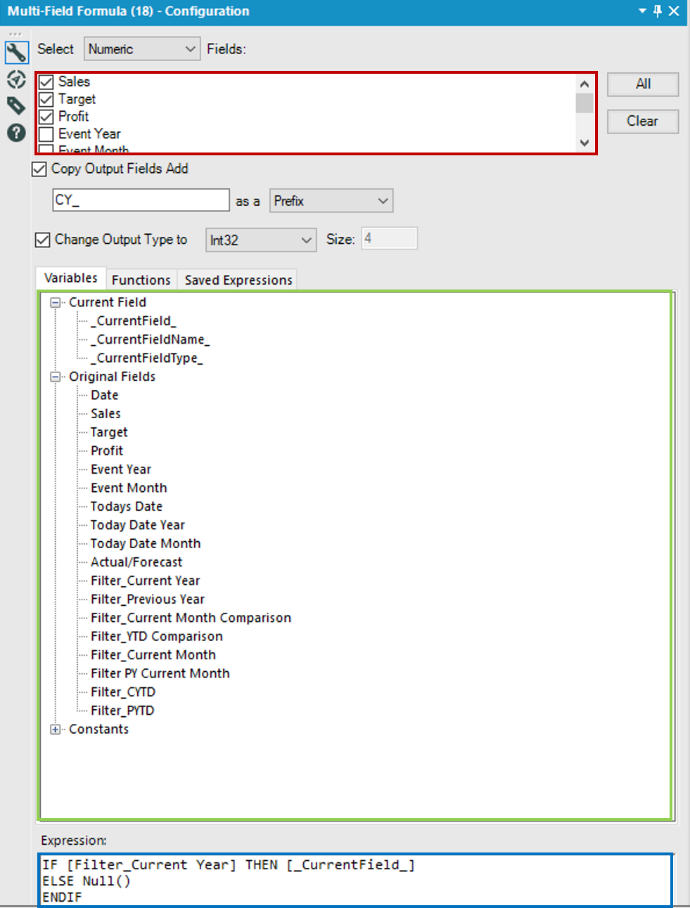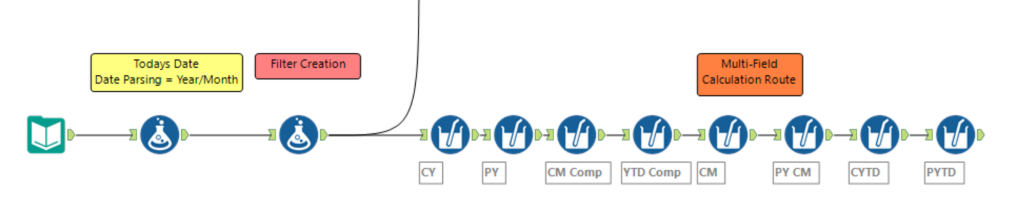×

Data

# Building Filters in Alteryx for Tableau, Part 2//

This post continues from Building Filters in Alteryx for Tableau, Part 1. In this, the foundation filter calculations were built. I advise going through Part 1 before looking at this section. If you would like to follow on with the workflow, it is possible to download this from the first blog post.

This post will be split into two alternative workflows where the filter calculations will be applied to a single measure or multiple measures.

Picking either option depends on the data. If there is only one value that needs to be manipulated, then the single-field calculation is obviously the more time efficient route. Some ideas that should be considered when choosing which route to go down are:

• How many fields do you want to calculate?
• Will more metrics be added to the data that need the same calculations?

If there are many metrics that need the same calculations, or more metrics need to be added to the data set, then the multi-field route should be taken.

## Section 1: Single-Metric Calculations

This will apply the filter calculations to one measure (Sales) in the workbook. The data will only return the value when the relevant metrics are True.  In this case, Sales will be the only metric we are using. Examples of the outputs are YTD Sales, Current Month Sales and Running Total Sales.

An IF statement will be written in Alteryx using the Formula Tool for each of the Filters calculations created in the previous blog post. The returning values will return sales values for when the relevant filter is True and NULL when False.

### Step 1:  CY_Sales

`IF [Filter_Current Year] THEN [Sales] ELSE Null() ENDIF`

### Step 2:  PY_Sales

`IF [Filter_Previous Year] THEN [Sales] ELSE Null() ENDIF`

### Step 3:  CM_Comparison Sales

`IF [Filter_Current Month Comparison] THEN [Sales] ELSE Null() ENDIF`

### Step 4:  YTD_Comparison Sales

`IF [Filter_YTD Comparison] THEN [Sales] ELSE Null() ENDIF`

### Step 5:  CM_Sales

`IF [Filter_Current Month] THEN [Sales] ELSE Null() ENDIF`

### Step 6:  PY_CM Sales

`IF [Filter PY Current Month] THEN [Sales] ELSE Null() ENDIF`

### Step 7:  CYTD_Sales

`IF [Filter_CYTD]  THEN [Sales] ELSE Null() ENDIF`

### Step 8:  PYTD_Sales

`IF [Filter_PYTD] THEN [Sales] ELSE Null() ENDIF`

### Step 9 (Optional)

It is possible to remove the filter calculations created in Part 1. Now that the required sales calculations have been created, these will be passed through the workflow into the Tableau workbook, meaning the filter calculations are no longer required. Below is a screenshot of the single measure workflow:## Section 2: Multi-Field Calculations

Multi-field calculations are an alternative approach when applying the filter calculations to the data values. This approach is suitable when there are several measures that require the same manipulations. These are created using a Multi-Field Formula Tool in Alteryx. Again, an IF statement is used in conjunction with the filter calculations created in the first blog post. However, instead of Sales being included in the formula, the value _CurrentField_ is used.

_CurrentField_ represents the fields that are selected in the Multi-Field Formula Tool. These are selected in the red box in the adjacent image. In this example, it represents the fields Sales, Target and Profit. This alternative workflow allows the users to calculate multiple measures using one formula.

The statement is almost identical to the single-metric calculation approach. However, instead of a specific metric being included (Sales), the _CurrentField_ variable is included. What this represents is dependent on the fields checked at the top of the configuration panel.An example of how this tool works is the following: A user is looking to return only the Current Year (CY) values for each of the three fields mentioned above. The user is looking to calculate the CY values for Sales, Target and Profit, so these three values will be checked in the configuration box. A prefix of “CY_” allows the data to have a similar naming structure for the three new values being calculated. In the drop-down menu below, it is possible to click and drag the required variables into the expression box. The variables under the header Current Field refer to the fields select in the red box. The header Original Fields refers to all the fields in the dataset. All the fields can be dragged into the expression box. In this example, the field Filter_Current Year and _CurrentField_ are used in the formula. Filter_Current Year will be replaced for each of the relevant filter calculations. See Steps 1-8.

Note: the Multi-Field Formula Tool can only hold one formula per tool, whereas the Formula Tool can hold a number of formulas in one instance of the tool.

### Step 1:  CY_value

`IF [Filter_Current Year] THEN [_CurrentField_] ELSE Null() ENDIF`

### Step 2:  PY_value

`IF [Filter_Previous Year] THEN [_CurrentField_] ELSE Null() ENDIF`

### Step 3:  CM_Comparison value

`IF [Filter_Current Month Comparison] THEN [_CurrentField_] ELSE Null() ENDIF`

### Step 4:  YTD_Comparison value

`IF [Filter_YTD Comparison] THEN [_CurrentField_] ELSE Null()ENDIF`

### Step 5:  CM_value

`IF [Filter_Current Month] THEN [_CurrentField_] ELSE Null() ENDIF`

### Step 6:  PY_CM value

`IF [Filter PY Current Month] THEN [_CurrentField_] ELSE Null() ENDIF`

### Step 7:  CYTD_value

`IF [Filter_CYTD] THEN [_CurrentField_] ELSE Null() ENDIF`

### Step 8:  PYTD_value

`IF [Filter_PYTD] THEN [_CurrentField_] ELSE Null() ENDIF`

### Step 9 (Optional)

It is possible to remove the filter calculations created in Part 1. Now that the required calculations have been created, these will be passed through the workflow into the Tableau workbook, meaning the filter calculations are no longer required. Below is a screenshot of the multi-measure workflow:In this example, due the simplicity of the data, the number of columns becomes far larger than the number of rows. As I mentioned at the start of the blog, the main takeaway is to give the user knowledge of how to pass the calculations from Tableau to Alteryx. Not all the calculations will be relevant to the user as the filter calculations will be chosen based on what is needed.

### The Latest

• ##### Snowflake External Tables: Connect Efficiently to Your Data Lake

Building Filters in Alteryx for Tableau, Part 2 This post continues from Building Filters in Alteryx for Tableau, Part 1. In this, the foundation filter calculations were built. I ...
Building Filters in Alteryx for Tableau, Part 1 Recently, I was required to prepare data in Alteryx and output it into a .Hyper file to create a Tableau workbook. The data that was ...

See more from this author →

InterWorks uses cookies to allow us to better understand how the site is used. By continuing to use this site, you consent to this policy. Review Policy OK

×

Interworks GmbH
Ratinger Straße 9
40213 Düsseldorf
Germany
Geschäftsführer: Mel Stephenson

Kontaktaufnahme: markus@interworks.eu
Telefon: +49 (0)211 5408 5301

Amtsgericht Düsseldorf HRB 79752
UstldNr: DE 313 353 072